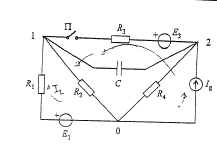# Voltage generators in open edges and Thevenin's generator

• irrationally

## Homework StatementI hope you can see the picture clearly. Someone drew lines over it,so please try to ignore that.
The problem here is to determine the current of ideal current source Ig, if following parameters are known:
-E1=50V, E3=30V
-R1=200Ω, R2=500Ω, R3=3kΩ, R4= 10 kΩ
-C=0.7 μF
-Charge flow trough the edge with capacitor after closing the switch Π is Q12 = 0.213 mC.

## Homework Equations

-Thevenin and Norton theorem.
-Ohm's law
-Millman's theorem

## The Attempt at a Solution

My attempt is to tranform the current generator to a voltage generator and then find the voltage U12. Then i would find Thevenin's generator and equivalent resistance and get a simple circuit. What to do after closing the switch. The biggest problem here is not exactly related to this problem - How does a voltage generator in an open edge affect the rest of the circut? Do we need to take it into calculations? How to set Kirchoff's second law equations? Are resistors in open edges included into calculating equivalent resistance for Thevenin's generator? These problems arise because my base knowledge of circuits is weak. :L:L:L

Does the problem statement specifically require you to use Thevenin's Theorem here? I can where nodal analysis might be advantageous.

Regarding open branches (E3 and R3 in your diagram when the switch is open), they have no effect on the circuit. Current cannot flow without a closed path. You may ignore them unless you need the potential at the open switch.

No, it does not. I'm allowed to solve it any way i want, but i mentioned Thevenin's theorem since i have confusions related to it and i tried using it. Any solution is welcome ;)

No, it does not. I'm allowed to solve it any way i want, but i mentioned Thevenin's theorem since i have confusions related to it and i tried using it. Any solution is welcome ;)
I see. Well, you could use Thevenin, but it may be more effort than necessary. Your choice.

What's your overall strategy for finding the current value?

Well first i find the Thevenin's EMF ( in function of Ig). Then i close the switch and now the voltage of the edge with R3 is equal to the voltage of the capacitor when the switch is open + Δq*C. There is no current flow trough the capacitor so we are left with a simple circuit with elements : E1, R5, Rt, Et(in function of Ig). From here , I hoped to somehow get the Et, but i am kinda stuck.

Well first i find the Thevenin's EMF ( in function of Ig). Then i close the switch and now the voltage of the edge with R3 is equal to the voltage of the capacitor when the switch is open + Δq*C. There is no current flow trough the capacitor so we are left with a simple circuit with elements : E1, R5, Rt, Et(in function of Ig). From here , I hoped to somehow get the Et, but i am kinda stuck.
Yeah, you probably want to start by finding expressions for the two steady-state voltages that will appear across the capacitor (both will involve Ig and some constants). Then you can use your change in capacitor voltage Δq*C to relate them. Essentailly that means find the potentials at nodes 1 and 2 for both cases.

Okay, i can find potentials at nodes 1 and 2 in the first case easily. But what do i do in the second case? How do I treat capacitor when using node potential method for example? My idea is that that branch doesn't contribute to overall current entering the node 0 or 1 , so it can be omitted in calculations. Is this correct?

Okay, i can find potentials at nodes 1 and 2 in the first case easily. But what do i do in the second case? How do I treat capacitor when using node potential method for example? My idea is that that branch doesn't contribute to overall current entering the node 0 or 1 , so it can be omitted in calculations. Is this correct?
Right. At steady state no current flows through the capacitor: it looks like an open circuit.

Oh well this then becomes a very simple problem. Thanks so much.